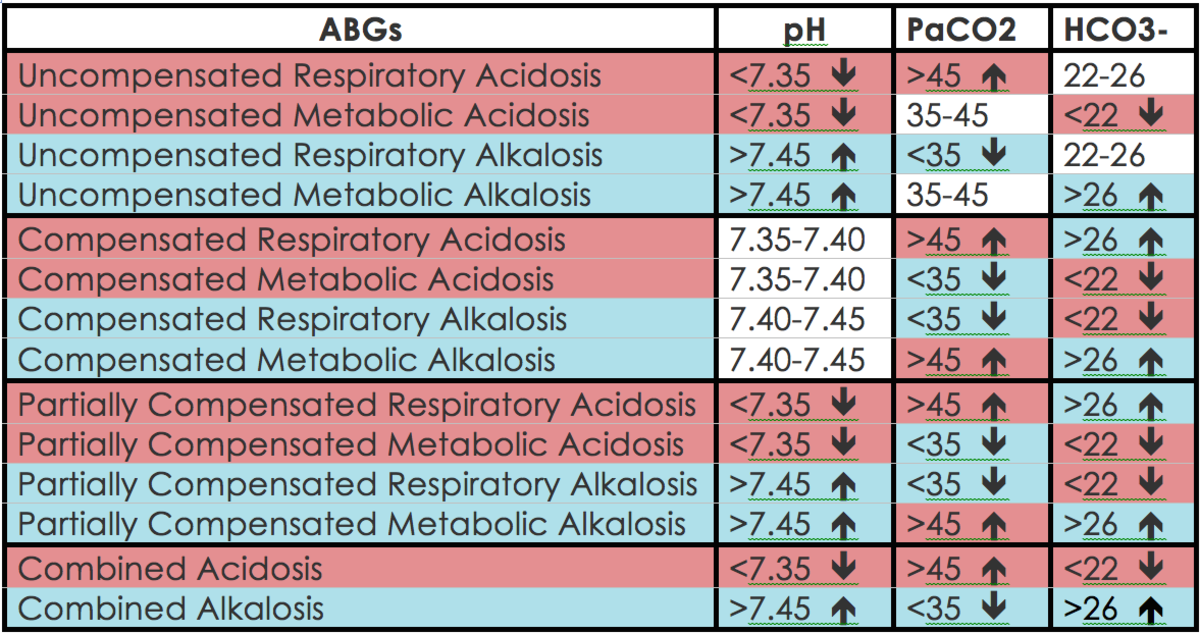# Arterial Blood Gases (ABGs): Simplified

To understand arterial blood gases (ABGs), you must first have a basic understanding of blood pH. Normal blood pH is 7.35 to 7.45. This is the safe range at which oxygen delivery to the tissues and metabolic processes can take place.

pH is classified as:

• Neutral (7.35 to 7.45)
• Acidotic (less than 7.35)
• Alkalotic (greater than 7.45)

Blood pH is regulated by the lungs via carbon dioxide (PaCO2) and the kidneys via sodium bicarbonate (HCO3-).

• If the pH is out of range due to the PaCO2, it is classified as "respiratory."
• If the pH is out of range due to the HCO3-, it is classified as "metabolic."
• If both the PaCO2 and HCO3- are contributing to the pH imbalance, it is classified as "combined."

## Respiratory vs. Metabolic

Now that you know that the lungs and kidneys contribute to blood pH, let's discuss normal ranges.

Normal blood PaCO2 range is 35-45 mmHg.

• PaCO2 greater than 45 mmHg this is classified as acidotic.
• PaCO2 less than 35 mmHg this is classified as alkalotic.
• Notice that the lower range is alkalosis and the higher range is acidosis*

------------------------------------------------------------------------------

If PaCO2 causes the pH imbalance, it is considered "respiratory acidosis/alkalosis."

Examples:

• pH = 7.50
• PaCO2 = 32 mmHg
• HCO3- = 24 mmHg

Interpretation: Respiratory alkalosis (pH = alkalotic range, PaCO2 = alkalotic range, HCO3- = normal)

• pH = 7.29
• PaCO2 = 50 mmHg
• HCO3- = 23 mmHg

Interpretation: Respiratory acidosis (pH = acidotic range, PaCO2 = acidotic range, HCO3- = normal)

------------------------------------------------------------------------------

Normal blood HCO3- range is 22-26 mmHg.

• HCO3- less than 22 mmHg is classified as acidotic.
• HCO3- greater than 26 mmHg is classified as alkalotic.

If HCO3- causes the pH imbalance, it is considered "metabolic acidosis/alkalosis."

Scroll to Continue

Examples:

• pH = 7.51
• PaCO2 = 42 mmHg
• HCO3- = 30 mmHg

Interpretation: Metabolic alkalosis (pH = alkalotic range, PaCO2 = normal range, HCO3- = alkalotic range)

• pH = 7.30
• PaCO2 = 40 mmHg
• HCO3- = 18 mmHg

Interpretation: Metabolic acidosis (pH = acidotic range, PaCO2 = normal range, HCO3- = acidotic range)

------------------------------------------------------------------------------

If all three values (pH, PaCO2, and HCO3-) are ALL out of range it is classified as "combined acidosis/alkalosis"

Examples:

• pH = 7.49
• PaCO2 = 32 mmHg
• HCO3- = 30 mmHg

Interpretation: Combined alkalosis (pH = alkalotic range, PaCO2 = alkalotic range, HCO3- = alkalotic range)

• pH = 7.26
• PaCO2 = 52 mmHg
• HCO3- = 16 mmHg

Interpretation: Metabolic acidosis (pH = acidotic range, PaCO2 = acidotic range, HCO3- = acidotic range)

## Full and Partial Compensation

Now that we've discussed the basics of acid-base balance and it's effect on pH, let's discuss compensation. When pH is out of range for long enough, the system that is not responsible for the imbalance will try to compensate.

Here are some hard and fast rules for interpreting compensation:

• If all three values are out of range (pH, PaCO2, and HCO3-) it is classified as "partially compensated."
• If the pH is in normal range, but PaCO2, and HCO3- are out of range it is classified as "fully compensated."

------------------------------------------------------------------------------

Here is a common example:

You have a chronic COPD patient. Due to the disease process, they are naturally retaining CO2. Their ABG may look something like this:

• pH: 7.36
• PaCO2: 50 mmHg
• HCO3-: 27 mmHg

Notice that pH is in normal range, but PaCO2 and HCO3- are out of range. The PaCO2 is acidotic and pH is on the acidotic side of normal. This means it is respiratory acidosis, BUT it is "fully compensated" by the metabolic system due to the pH falling in normal range. So you would classify it as "fully compensated respiratory acidosis."

------------------------------------------------------------------------------

Another common example:

A diabetic patient in ketoacidosis might have an ABG that looks something like this:

• pH: 7.25
• PaCO2: 28 mmHg
• HCO3-: 16 mmHg

Because this is an acute condition, the PaCO2 has started trying to compensate for the imbalance, but hasn't had time to "fully compensate." This would be considered "partially compensated metabolic acidosis."

## The Ultimate ABG Interpretation Chart (Easy!)Use this chart either by color, by value, or by the arrows (like you would see in lab results).

## ROME

A popular technique using the arrows is "ROME: respiratory opposite, metabolic equal.

So, for example, you would recognize respiratory acidosis by pH ↓ and PaCO2 ↑. Metabolic acidosis would be recognized by pH ↓ and HCO3- ↓.

I personally ignore arrows and first look at the pH, is it acidotic/alkalotic? If so, I match it to either PaCO2 or HCO3-, whichever is acidotic/alkalotic (like matching colors on the table). That simple.

I hope you enjoyed this brief overview of ABG analysis. This was a very basic overview with a few examples to supplement the material. My goal in writing this was to try and help others understand ABG interpretations, as they can seem very overwhelming. When I received this information in class it was a whirlwind of confusion. I tried to present it in a way that helped me understand in hopes that it would do the same for you.